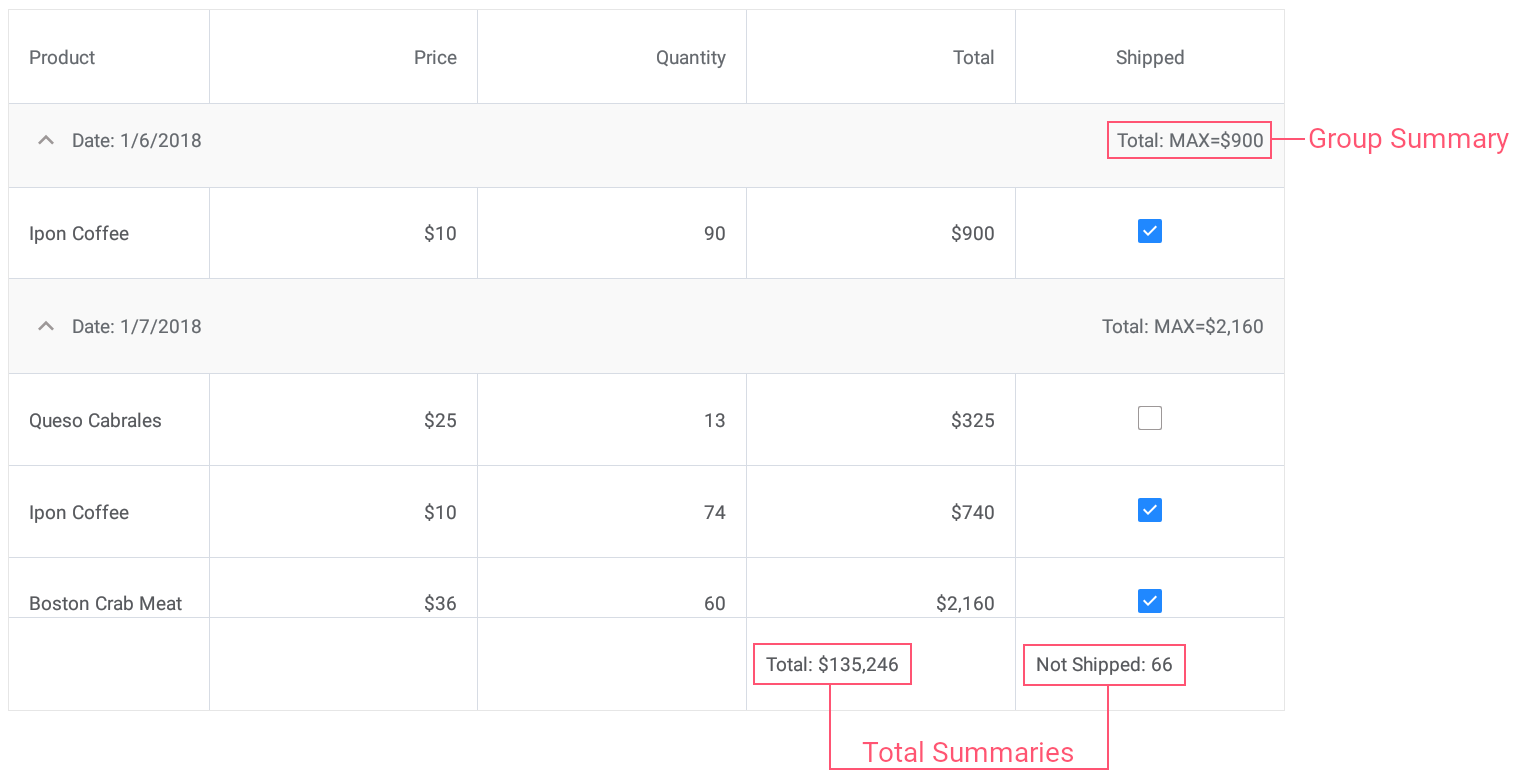# GridColumnSummary Class

A data summary item.

Namespace: DevExpress.XamarinForms.DataGrid

Assembly: DevExpress.XamarinForms.Grid.dll

## Declaration

``````public class GridColumnSummary :
BindableObject,
IXtraSerializableLayoutEx``````

## Remarks

DataGridView allows you to display summary information about individual data columns or groups of rows. For example, you can display the sum of values, the number of records, the minimum or maximum value, etc.

The grid supports two summary types: total summaries (calculated over all rows in a column and displayed below this column) and group summaries (calculated over all rows in a group and displayed in a group row).

To create a total or group summary, add a GridColumnSummary object to the DataGridView.TotalSummaries or DataGridView.GroupSummaries collection, respectively. Use the following properties to adjust summary settings:

## Example

This example demonstrates how to use predefined aggregate functions and custom rule to calculate group and total summaries for grid columns.• Set the group summary to display the maximum Total value for each group of records.
• Set the total summary to calculate the sum of values in the Total column.
• Set the custom total summary to count the number of orders whose value in the Shipped column is false (to count orders that are not shipped).
``````<dxg:DataGridView x:Name="grid" ItemsSource="{Binding Orders}"
CalculateCustomSummary="OnCalculateCustomSummary">
<!-- ... -->
<dxg:DataGridView.GroupSummaries>
<dxg:GridColumnSummary FieldName="Total" Type="Max"/>
</dxg:DataGridView.GroupSummaries>

<dxg:DataGridView.TotalSummaries>
<dxg:GridColumnSummary FieldName="Total" Type="Sum"
DisplayFormat="Total: {0:C0}"/>
<dxg:GridColumnSummary FieldName="Shipped" Type="Custom"
DisplayFormat="Not Shipped: {0}"/>
</dxg:DataGridView.TotalSummaries>
</dxg:DataGridView>
``````
``````int count;
// ...

void OnCalculateCustomSummary(object sender, CustomSummaryEventArgs e) {
if (e.FieldName.ToString () == "Shipped")
if (e.IsTotalSummary){
if (e.SummaryProcess == CustomSummaryProcess.Start) {
count = 0;
}
if (e.SummaryProcess == CustomSummaryProcess.Calculate) {
if (!(bool)e.FieldValue)
count++;
e.TotalValue = count;
}
}
}
``````

## Inheritance

Object
GridColumnSummary